Courses

# Test: Kinematics of Flow & Ideal Flow - 3

## 15 Questions MCQ Test Topicwise Question Bank for Mechanical Engineering | Test: Kinematics of Flow & Ideal Flow - 3

Description
This mock test of Test: Kinematics of Flow & Ideal Flow - 3 for Mechanical Engineering helps you for every Mechanical Engineering entrance exam. This contains 15 Multiple Choice Questions for Mechanical Engineering Test: Kinematics of Flow & Ideal Flow - 3 (mcq) to study with solutions a complete question bank. The solved questions answers in this Test: Kinematics of Flow & Ideal Flow - 3 quiz give you a good mix of easy questions and tough questions. Mechanical Engineering students definitely take this Test: Kinematics of Flow & Ideal Flow - 3 exercise for a better result in the exam. You can find other Test: Kinematics of Flow & Ideal Flow - 3 extra questions, long questions & short questions for Mechanical Engineering on EduRev as well by searching above.
QUESTION: 1

### In a two-dimensional flow, the velocity components in x and y directions in terms of stream function (ψ) are

Solution:

For stream function:
Velocity component in x-direction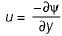Velocity component in y-direction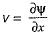QUESTION: 2

### If φ is the velocity potential function in 2-D flow field, then the velocity components u and v are defined as

Solution:

For velocity potential function
Velocity component in x direction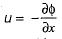Velocity component in y-direction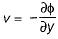QUESTION: 3

### The convective acceleration of fluid in the x-direction is given by

Solution: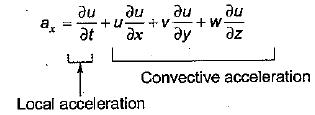QUESTION: 4

A stream line is a fine

Solution:

Stream line is an imaginary line drawn in space such that a tangent drawn to it at any point gives velocity at that point.

QUESTION: 5

A steady incompressible flow is given by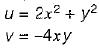Q. What is the convective acceleration along x-direction at point (1, 2)?

Solution:

Convective acceleration along x direction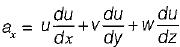for 2-D flow (w=0)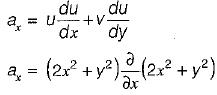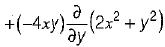ax = (2x2 + y2)(4x) - 4xy(2y)
ax = 8x3 + 4xy2 - 8xy2
at (1-2)
ax = 8 + 16 - 32 = - 8 unit

QUESTION: 6

Match List-I with List-ll and select the correct answer using the codes given below the lists:
List-I
A. Stream line
B. Streak line
C. Path line
D. Equipotential lines
List-II
1. Tracing of motion of any fluid particle
2. Tracing of motion of different fluid particles
3. Identification of location of number of fluid particles
4. Orthogonal to streak lines
5. Location of equal piezometric head
Codes:
A B C D
(a) 2 3 4 5
(b) 3 2 1 4
(c) 1 2 4 3
(d) 2 3 1 5

Solution:

Stream Line — Curves drawn tangential to the velocity vector at all points in the flow field at any instance of time. They represent Eulerian approach for flow field.
Streak Line — It is locus of the temporary location of all the particles that have passed through a fixed point in the flow field.

QUESTION: 7

The stream function for a two-dimensional flow is given by ψ = 2xy. The velocity at (2, 2) is

Solution: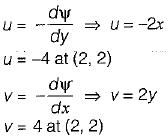Resultant =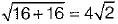QUESTION: 8

You are asked to evaluate assorted fluid flows for their suitability in a given laboratory application. The following three flow choices, expressed in terms of 2-D velocity field in the xy plane, are made available
P. u = 2y,v = -3x
Q. u = 3xy, v = 0
R. u = -2x , v = 2 y

Which flow(s) should be recommended when the application requires the flow to be incompressible and irrotational

Solution:
QUESTION: 9

The continuity equation in differential form is

Solution:

Continuity equation
ρAV = constant
By differentiating the equation
Av.dρ + ρvdA + ρAdV = 0
By dividing pAv, we get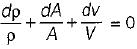QUESTION: 10

Given φ = 3 xy and ψ = 3/2(y2 - x2) , the discharge passing between the stream tines through the point (1,3) and (3,3) is

Solution:

Note that difference between two stream line is discharge per unit width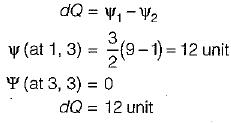QUESTION: 11

If the stream function is given by ψ = 3xy then the velocity at a point (2,3) will be

Solution: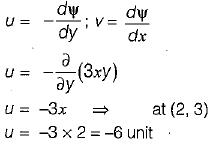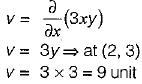Resultant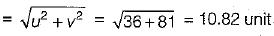QUESTION: 12

An open circular cylinder 1.2 m height is filled with a liquid to its top. The liquid is given a rigid body rotation about the axis of the cylinder and the pressure at the centre line at the bottom surface is found to be 0.6 m of liquid. What is the ratio of volume of liquid spilled out of the cylinder to the original volume

Solution: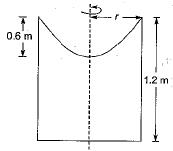original volume of cylinder = πr2h
volume of liquid spilled out = 1/2 πr2h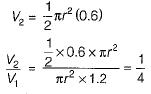QUESTION: 13

In a two dimensional velocity field with velocities u and v along the x and y directions respectively the convective acceleration along the x-direction is given by

Solution:
QUESTION: 14

A type of flow in which the fluid particle while moving in the direction of flow rotates about their centre is known as

Solution:
QUESTION: 15

For the continuity equation given by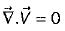to be valid, where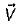is the velocity vector, which one of the following is a necessary condition?

Solution:

Track your progress, build streaks, highlight & save important lessons and more!

### Similar Content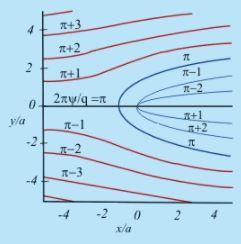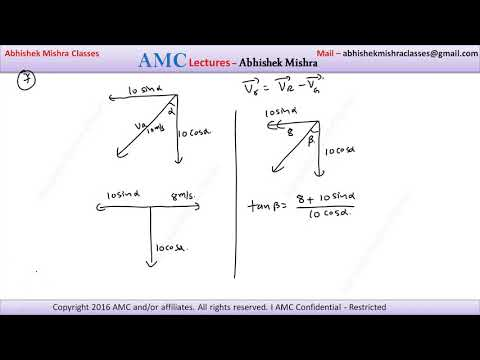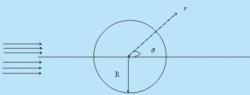### Related tests